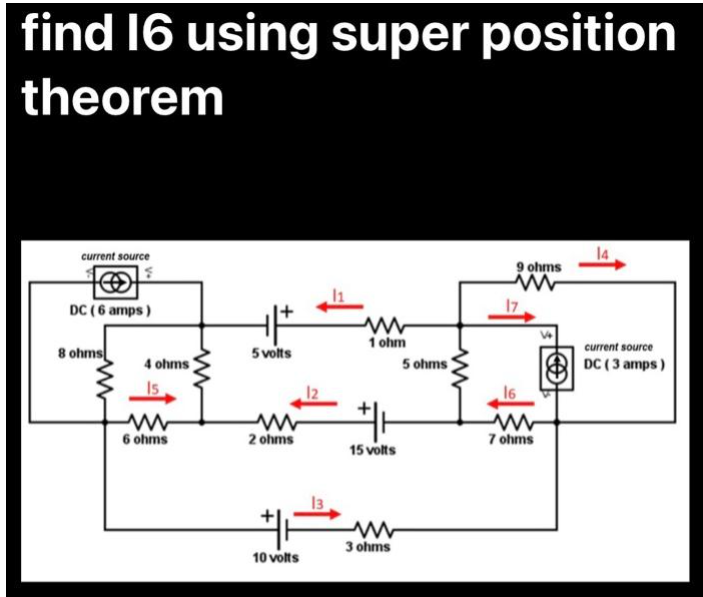# Question How does Superposition Theorem differ from all the other network laws? What is/are its limitation/s in terms of applicability? find 16 using super position theorem 14 current source 9 ohms ( www DC (6 amps) 1 ohm current source DC (3 amps) #H 15 volts 3 ohms 8 ohms www 4 ohms) Is www 6 ohms +|+ 5 volts 2 ohms 10 volts 13 5 ohms ww 17 16 7 ohmsE8KETV The Asker · Electrical Engineering

How does Superposition Theorem differ from all the other network laws? What is/are its limitation/s in terms
of applicability?Transcribed Image Text: find 16 using super position theorem 14 current source 9 ohms ( www DC (6 amps) 1 ohm current source DC (3 amps) #H 15 volts 3 ohms 8 ohms www 4 ohms) Is www 6 ohms +|+ 5 volts 2 ohms 10 volts 13 5 ohms ww 17 16 7 ohms
More
Transcribed Image Text: find 16 using super position theorem 14 current source 9 ohms ( www DC (6 amps) 1 ohm current source DC (3 amps) #H 15 volts 3 ohms 8 ohms www 4 ohms) Is www 6 ohms +|+ 5 volts 2 ohms 10 volts 13 5 ohms ww 17 16 7 ohms